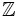# Characteristic of a ring

Let$R$ be a commutative unital ring. Consider the natural homomorphism from$\Z$ to$R$ that sends$1 \in \Z$ to$1 \in R$. The positive element that generates the kernel of this map, is termed the characteristic of$R$.
In other words, the characteristic of$R$ is the smallest number$n$ such that$1 + 1 + \ldots + 1 = 0$ when$1$ is written$n$ times.